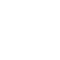# Machine learning workout

\$20.00

Holger Ortega Martínez

In the crowded population of texts on Machine Learning, the present book is unique in the sense that it keeps the spirit of the books on basic subjects, like Calculus: its core is made up of many problems with answers, so that the reader can exercise and detect any misunderstanding on time.

The book, then, es not for passive reading. It is meant for learning through exercising. Hard workout. Therefore, each chapter presents a set of exercises to be solved “by hand” (think of a desk check) and a strong set of  programming tasks to be solved using MATLAB.

Being intended for undergraduates, the book does not dive into deep mathematical waters. It is aimed instead to a deep comprehension of the concepts: the mechanics of the algorithms, the structure and geometric representation of the data, the precise evaluation of the results. All by doing it yourself, like in the good old days.

I GENERALITIES
CH. 1 MATRICES AND MATLAB
1.1 Theoretical Briefing
1.2 Exercises
1.3 Practical in MATLAB
CH. 2 DEFINITIONS AND DATA REPRESENTATION
2.1 Theoretical Briefing
2.2 Exercises
2.3 Practical in MATLAB
CH. 3 CLASSIFICATION PROBLEMS
3.1 Theoretical Briefing
3.2 Exercises
3.3 Practical in MATLAB
CH. 4 REGRESSION PROBLEMS
4.1 Theoretical Briefing
4.2 Exercises
4.3 Practical in MATLAB
II REGRESSION
CH. 5 LINEAR REGRESSION
5.1 Theoretical Briefing
5.2 Exercises
5.3 Practical in MATLAB
CH. 6 OVERFITTING AND UNDERFITTING: VISUALISATION
6.1 Theoretical Briefing
6.2 Exercises
6.3 Practical in MATLAB
III HISTORIC ALGORITHMS
CH. 7 MCCULLOCH-PITTS NEURON
7.1 Theoretical Briefing
7.2 Exercises
7.3 Practical in MATLAB
CH. 8 PERCEPTRON
8.1 Theoretical Briefing
8.2 Exercises
8.3 Practical in MATLAB
9.1 Theoretical Briefing
9.2 Exercises
9.3 Practical in MATLAB
IV CLASSIFICATION ALGORITHMS
CH. 10 NEURAL NETWORKS: FORWARD PROPAGATION
10.1 Theoretical Briefing
10.2 Exercises
10.3 Practical in MATLAB
CH. 11 NEURAL NETWORKS: VALIDATION AND TEST
11.1 Theoretical Briefing
11.2 Exercises
11.3 Practical in MATLAB
CH. 12 NEURAL NETWORK PATTERN RECOGNITION APP
12.1 Theoretical Briefing
12.2 Exercises
12.3 Practical in MATLAB
CH. 13 SUPPORT VECTOR MACHINES
13.1 Theoretical Briefing
13.2 Exercises
13.3 Practical in MATLAB
CH. 14 K-NEAREST NEIGHBOURS
14.1 Theoretical Briefing
14.2 Exercises
14.3 Practical in MATLAB
V PROBLEMS IN IMPLEMENTATION TIME
CH. 15 FEATURE SCALING
15.1 Theoretical Briefing
15.2 Exercises
15.3 Practical in MATLAB
CH. 16 LEARNING CURVES
16.1 Theoretical Briefing
16.2 Exercises
16.3 Practical in MATLAB
CH. 17 PRINCIPAL COMPONENT ANALYSIS
17.1 Theoretical Briefing
17.2 Exercises
17.3 Practical in MATLAB
17.4 PRACTICAL IN MATLAB
VI UNSUPERVISED LEARNING
CH. 18 K-MEANS ALGORITHM
18.1 Theoretical Briefing
18.2 Exercises
18.3 Practical in MATLAB